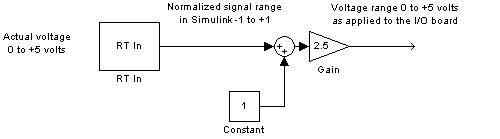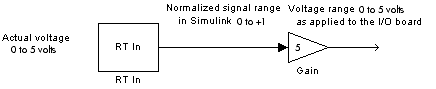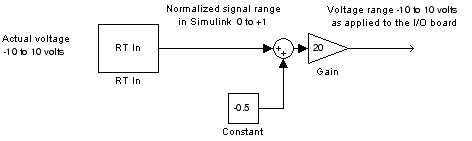## Use Analog I/O Drivers

Control systems have unique requirements for I/O devices used with Simulink® Desktop Real-Time™ applications. For information about writing custom I/O device drivers to work with Simulink Desktop Real-Time applications, see Custom I/O Driver Basics.

### Configure I/O Driver Characteristics

Simulink Desktop Real-Time applications use I/O boards provided by many hardware vendors. These boards are often used for data acquisition independently of Simulink Desktop Real-Time software. In such environments, board manufacturers usually provide their own I/O device drivers for data acquisition purposes. This use differs significantly from the behavior of drivers provided with Simulink Desktop Real-Time software.

In data acquisition applications, data is often collected in a burst or frame consisting of many points, possibly 1,000 or more. The burst of data becomes available once the final point is available. This approach is not suitable for automatic control applications, because it results in unacceptable latency for most of the data points.

In contrast, drivers used by Simulink Desktop Real-Time applications capture a single data point at each sample interval. The software gives considerable effort to minimize the latency between collecting a data point and using the data in the control system algorithm. Sometimes a board can specify a maximum sample rate (for data acquisition) higher than the rates achievable by Simulink Desktop Real-Time applications. For data acquisition, such boards usually acquire data in bursts and not in the point-by-point fashion required by control systems.

Simulink Desktop Real-Time software allows you to normalize I/O signals internal to the block diagram. Generally, inputs represent real-world values such as angular velocity, position, temperature, and pressure. This ability to normalize signals allows you to

• Apply your own scale factors

• Work with meaningful units without having to convert from voltages

When using an Analog Input block, you select the range of the external voltages that the board receives, and you select the block output signal. For example, you could set the voltage range to `0 to +5 V`, and the block output signal as `Normalized unipolar`, ```Normalized bipolar```, `Volts`, or `Raw`.

If you prefer to work with units of voltage within your Simulink block diagram, you can select `Volts`.

To apply your own scaling factor, select ```Normalized unipolar``` or `Normalized bipolar`, add a Gain block, and add an offset to convert the value to a meaningful value in your model.

If you prefer nonrounded integer values from the analog-to-digital conversion process, you can select `Raw`.

#### `0 to +5 Volts` and `Normalized bipolar`

From the Input range list, select `0 to +5 V`, and from the `Block output signal `list, select `Normalized bipolar`. This example converts a normalized bipolar value to volts, but you could also easily convert directly to another parameter in your model.

```0 to 5 volts --> ([-1 to 1] normalized + 1) * 2.5 ```

In your block diagram, you can convert the normalized value to volts as follows.#### `0 to +5 V` and `Normalized unipolar`

From the `Input range` list, select ```0 to +5 V```, and from the `Block output signal `list, select `Normalized unipolar`. This example converts a normalized unipolar value to volts, but you could also easily convert directly to another parameter in your model.

```0 to 5 volts --> ([0 to 1] normalized * 5.0 ```

In your block diagram, you can convert the normalized value to volts as follows.#### `-10 to +10 V` and `Normalized bipolar`

From the `Input range` list, select ```-10 to +10 V```, and from the `Block output signal` list, select `Normalized bipolar`. This example converts a normalized bipolar value to volts, but you could also easily convert directly to another parameter in your model.

```-10 to 10 volts --> [-1 to +1] normalized * 10 ```

In your block diagram, you can convert the normalized value to volts as follows.#### `-10 to +10 V` and `Normalized unipolar`

From the `Input range` list, select ```-10 to +10 V```, and from the `Block output signal` list, select `Normalized unipolar`. This example converts a normalized bipolar value to volts, but you could also easily convert directly to another parameter in your model.

```-10 to 10 volts --> ([0 to 1] normalized - 0.5) * 20 ```

In your block diagram, you can convert the normalized value to volts as follows.#### Normalize Scaling for Analog Outputs

For example, assume that the voltage range on the D/A converter is set to `0 to +5 volts` and the ```Block input signal``` is selected as `Normalized bipolar`. With this configuration, a Simulink signal of amplitude `-1` results in an output voltage of `0` volts. Similarly, a Simulink signal of amplitude `+1` results in an output voltage of `+5` volts.
For another example, assume that the voltage range on the D/A converter is set to `-10 to +10 volts` and the ```Block input signal``` is selected as `Normalized bipolar`. With this configuration, a Simulink signal of amplitude `-1` results in an output voltage of `-10` volts. Similarly, a Simulink signal of amplitude `+1` results in an output voltage of `+10` volts.